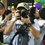# Do you understand?

Is $$2 + 2^2 + 2^3 + 2^4 + ... + 2^{10}$$ divisible by $$3$$?

It seems undetermined, because there were no threes, but let's try to pair it, extract it to find the threes.

$A = (2 + 2^2) + (2^3 + 2^4)+...+ (2^9 + 2^{10})$

$A = 2(1+2) + 2^3(1+2) + ... + 2^9(1+2)$

$A = 2 \times 3 + 2^3 \times 3 + ... + 2^9 \times 3$

$A = (2 +2^3 + ... + 2^9) \times 3$

Look! The series has x3! So A is divisible by 3.6 years, 6 months ago

This discussion board is a place to discuss our Daily Challenges and the math and science related to those challenges. Explanations are more than just a solution — they should explain the steps and thinking strategies that you used to obtain the solution. Comments should further the discussion of math and science.

When posting on Brilliant:

• Use the emojis to react to an explanation, whether you're congratulating a job well done , or just really confused .
• Ask specific questions about the challenge or the steps in somebody's explanation. Well-posed questions can add a lot to the discussion, but posting "I don't understand!" doesn't help anyone.
• Try to contribute something new to the discussion, whether it is an extension, generalization or other idea related to the challenge.

MarkdownAppears as
*italics* or _italics_ italics
**bold** or __bold__ bold
- bulleted- list
• bulleted
• list
1. numbered2. list
1. numbered
2. list
Note: you must add a full line of space before and after lists for them to show up correctly
paragraph 1paragraph 2

paragraph 1

paragraph 2

[example link](https://brilliant.org)example link
> This is a quote
This is a quote
    # I indented these lines
# 4 spaces, and now they show
# up as a code block.

print "hello world"
# I indented these lines
# 4 spaces, and now they show
# up as a code block.

print "hello world"
MathAppears as
Remember to wrap math in $$ ... $$ or $ ... $ to ensure proper formatting.
2 \times 3 $2 \times 3$
2^{34} $2^{34}$
a_{i-1} $a_{i-1}$
\frac{2}{3} $\frac{2}{3}$
\sqrt{2} $\sqrt{2}$
\sum_{i=1}^3 $\sum_{i=1}^3$
\sin \theta $\sin \theta$
\boxed{123} $\boxed{123}$

Sort by:

Yes it is divisible!!!!!!!!!!!!!

- 6 years, 6 months ago

An easier way to prove that it is divisible by $3$ is by using modular arithmetic. Let the given expression be $Z$.

$Z=\sum_{i=1}^{10} 2^i \equiv \sum_{i=1}^{10} (-1)^i\pmod{3}\\ \implies Z\equiv \left(\sum_{i=1}^5 (-1)^{2i}\right)+\left(\sum_{i=1}^{5} (-1)^{2i-1}\right)\pmod{3}\\ \implies Z\equiv \left(\sum_{i=1}^5 (1)\right)+\left(\sum_{i=1}^5 (-1)\right)\equiv \sum_{i=1}^5 (1-1)\pmod{3}\\ \implies Z\equiv \sum_{i=1}^5 (0)\pmod{3}\\ \implies Z\equiv 0\pmod{3}\\ \implies 3\mid Z$

where $a\mid b$ denotes that $a$ divides $b$, or in other words, $b$ is divisible by $a$.

- 6 years, 5 months ago

I'm an 7th grade student, so I just use original numbers :)) Sorry, can't understand.

- 6 years, 5 months ago

It's nothing much complicated. You can review the modular arithmetic wikis or ask me which part of it you can't understand?! I'd be more than glad to help you out. :)

- 6 years, 5 months ago

What is the i?

- 6 years, 5 months ago

$i$ is a counter variable in the summation. Suppose, you have the sum $S=2^1+2^2+2^3+\ldots+2^6$. You can write it as,

$S=\sum_{i=1}^6 2^i$

The initial and final values of $i$ are given in lower and upper sides of the "sigma" symbol and the value of $i$ gets incremented in each summation step.

- 6 years, 5 months ago